# TGS Revision Year 10 Physics

Specification for 1.1 motion- 6.2 Nuclear Radiation

?
• Created on: 04-05-13 11:50

## Forces and their effects: Motion

a) Scalars are quantities that have magnitude only. Vectors are quantities that have magnitude and an associated direction.

Candidates should be aware that distance, speed and time are examples of scalars and displacement, velocity, acceleration, force and momentum are examples of vectors.

b) If an object moves in a straight line, how far it is from a certain point can be represented by a distance– time graph.

c) The speed of an object can be determined from the gradient of a distance–time graph. If an object is accelerating its speed at any particular time can be determined by finding the gradient of the tangent of the distance–time graph at that time.

d) The velocity of an object is its speed in a given direction.

e) The velocity v of an object is given by the equation: v=s/where s is the displacement and t is the time taken.

1 of 42

## Motion

f) The acceleration a of an object is given by the equation:

a= v – u/t

where u is the initial velocity, v is the final velocity and t is the time taken.

g) The acceleration of an object can be determined from the gradient of a velocity–time graph.

h) The distance travelled by an object can be determined from the area under a velocity–time graph.

2 of 42

## Resultant Forces

a) Whenever two objects interact, the forces they exert on each other are equal and opposite.

b) A number of forces acting at a point may be replaced by a single force that has the same effect on the motion as the original forces all acting together. This single force is called the resultant force.

Candidates should be able to determine the resultant of opposite or parallel forces acting in a straight line and determine the resultant of two coplanar forces by scale drawing.

c) A resultant force acting on an object may cause a change in its state of rest or motion.

d) If the resultant force acting on a stationary object is:

• zero, the object will remain stationary
• not zero, the object will accelerate in the direction of the resultant force.
3 of 42

## Resultant Forces

e) If the resultant force acting on a moving object is:

• zero, the object will continue to move at the same speed and in the same direction
• not zero, the object will accelerate in the direction of the resultant force.

f) The relationship between force F, mass m and acceleration a is:

F = m x a

4 of 42

## Momentum

a) The relationship between momentum p, mass m and velocity v is:

p= m x v

b) In a closed system the total momentum before an event is equal to the total momentum after the event. This is called conservation of momentum.

Candidates may be required to complete calculations involving two objects. Examples of events are collisions and explosions.

c) The relationship between force F, change in momentum ^p and time t is:

F= ^p/t

Candidates should be able to use this relationship to explain safety features such as air bags, seat belts,gymnasium crash mats, cushioned surfaces for playgrounds and cycle helmets.

5 of 42

## Forces and Braking

a) When a vehicle travels at a steady speed the resistive forces balance the driving force.

Candidates should realise that most of the resistive forces are caused by air resistance.

b) The greater the speed of a vehicle the greater the braking force needed to stop it in a certain distance.

Candidates should understand that for a given braking force, the greater the speed, the greater the stopping distance.

c) The stopping distance of a vehicle is the sum of the distance the vehicle travels during the driver’s reaction time (thinking distance) and the distance it travels under the braking force (braking distance).

d) A driver’s reaction time can be affected by tiredness, drugs and alcohol.

Candidates should appreciate that distractions may affect a driver’s ability to reac

6 of 42

## Forces and Braking

e) When the brakes of a vehicle are applied, work done by the friction force between the brakes and the wheel reduces the kinetic energy of the vehicle and the temperature of the brakes increases.

f) A vehicle’s braking distance can be affected by adverse road and weather conditions and poor condition of the vehicle.

Candidates should understand that ‘adverse road conditions’ includes wet or icy conditions. Poor condition of the car is limited to the car’s brakes or tyres.

7 of 42

## Forces and terminal velocity

a) The faster an object moves through a fluid the greater the frictional force which acts on it.

b) An object falling through a fluid will initially accelerate due to the force of gravity. Eventually the resultant force will be zero and the object will move at its terminal velocity (steady speed).

Candidates should understand why the use of a parachute reduces the parachutist’s terminal velocity. Candidates should be able to draw and interpret velocity–time graphs for objects that reach terminal velocity, including a consideration of the forces acting on the object.

c) The relationship between weight W, mass m and gravitational field strength (acceleration of free fall) g is:

W = m x g

Candidates will not be expected to know the value of g.

8 of 42

## Forces and Energy

a) Work is done when a force causes an object to move through a distance.

b) The relationship between work done W, force F and distance d moved in the direction of the force is:

W = F x d

c) Energy is transferred when work is done.

d) Work done against frictional forces causes energy transfer by heating.

Candidates should be able to discuss the transfer of kinetic energy in particular situations, for example shuttle re-entry into the atmosphere or meteorites burning up in the atmosphere and braking systems on vehicles.

e) The relationship between power P, work done or energy transferred W and time t is:

P= W/t

9 of 42

## Forces and Energy

f) The relationship between gravitational potential energy Ep, mass m, gravitational field strength (acceleration of free fall) g and height h is:

Ep= m x g x h

Candidates should understand that when an object is raised vertically work is done against the gravitational force and the object gains gravitational potential energy.

g) The relationship between kinetic energy Ek, mass m and speed v is:

Ek= 1/2 x m x v2

Candidates should understand that an object of double the mass of another object travelling with the same speed will have double the kinetic energy. Candidates should understand that an object travelling at double the speed of another object with the same mass will have four times the kinetic energy. They should be able to apply this idea in the context of road safety.

10 of 42

## Waves: General Properties of Waves

a) Waves transfer energy and information without transferring matter.

b) In a transverse wave the oscillations are perpendicular to the direction of energy transfer.

c) In a longitudinal wave the oscillations are parallel to the direction of energy transfer.

Longitudinal waves show areas of compression and rarefaction.

d) Electromagnetic waves are transverse, sound waves are longitudinal and mechanical waves may be either transverse or longitudinal.

g) Waves may be described in terms of their frequency, wavelength, time period and amplitude.

Candidates should be able to explain the meaning of these terms.

h) The relationship between wave speed v, frequency f and wavelength is:

v = f x11 of 42

## The electromagnetic spectrum

a) Electromagnetic waves form a continuous spectrum and all types of electromagnetic wave travel at the same speed through a vacuum (space).

Candidates should know the order of electromagnetic waves within the spectrum, in terms of energy, frequency and wavelength. Candidates should appreciate that the wavelengths of the electromagnetic spectrum range from 10–15 m to 104 m and beyond.

b) Radio waves, microwaves, infrared and visible light can be used for communication.

c) Electromagnetic waves have many uses. For example:

• microwaves – mobile phones and satellite television
• infrared – remote controls
• visible light – photography
• ultraviolet – security marking
• X-rays – medical imaging
• gamma rays – sterilising surgical instruments and killing harmful bacteria in food.
12 of 42

## The electromagnetic spectrum

d) Exposure to electromagnetic waves can be hazardous. For example:

• microwaves – heating of body tissue
• infrared – skin burns
• ultraviolet – skin cancer and blindness
• X-rays – high doses kill cells
• gamma rays – genetic mutations.

e) X-rays are part of the electromagnetic spectrum. They have a very short wavelength, high energy and cause ionisation.

f) Properties of X-rays include:

• they affect a photographic film in the same way as light
• they are absorbed by metal and bone
• they are transmitted by healthy tissue.
13 of 42

## The electromagnetic spectrum

d) Exposure to electromagnetic waves can be hazardous. For example:

• microwaves – heating of body tissue
• infrared – skin burns
• ultraviolet – skin cancer and blindness
• X-rays – high doses kill cells
• gamma rays – genetic mutations.

e) X-rays are part of the electromagnetic spectrum. They have a very short wavelength, high energy and cause ionisation.

f) Properties of X-rays include:

• they affect a photographic film in the same way as light
• they are absorbed by metal and bone
• they are transmitted by healthy tissue.
14 of 42

## The electromagnetic spectrum

g) X-rays can be used to diagnose some medical conditions, for example in computed tomography (CT) scanning, bone fractures and dental problems. X-rays are also used to treat some conditions, for example in killing cancer cells.

h) The use of high energy ionising radiation can be dangerous, and precautions need to be taken to monitor and minimise the levels of radiation that people who work with it are exposed to.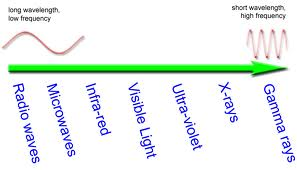15 of 42

## Sound and ultrasound

a) Sound waves are longitudinal waves and cause vibrations in a medium, which are detected as sound.

b) The range of human hearing is about 20 Hz to 20 000 Hz.

c) The pitch of a sound is determined by its frequency and loudness by its amplitude.

d) Sound waves can be reflected (echoes) and diffracted.

e) Ultrasound is acoustic (sound) energy, in the form of waves with a frequency above the human hearing range.

f) Electronic systems can be used to produce ultrasound waves, which have a frequency higher than the upper limit of hearing for humans.

g) Ultrasound waves are partially reflected when they meet a boundary between two different media. The time taken for the reflections to reach a detector can be used to determine how far away such a boundary is.

16 of 42

## Sound and ultrasound

h) The distances between interfaces in various media can be calculated using:

s = v x t

where v is wave speed and t is time taken.

Candidates may be required to use data from diagrams of oscilloscope traces.

i) Ultrasound waves can be used in medicine. Examples include pre-natal scanning and the removal of kidney stones.

17 of 42

## Reflection+Refraction and total internal reflectio

a) When waves are reflected the angle of incidence is equal to the angle of reflection.

b) The normal is a construction line perpendicular to the reflecting surface at the point of incidence.

c) The image produced in a plane mirror is virtual, upright and laterally inverted.

Candidates will be expected to be able to construct ray diagrams.

a) Light waves undergo a change of direction when they pass from one medium to another at an interface. This is called refraction.  When light enters a more dense medium it is refracted towards the normal. When light enters a less dense medium it is refracted away from the normal. Waves are not refracted if travelling along the normal. Waves are refracted due to change of speed. b) Refraction by a prism can lead to dispersion. f) Total internal reflection is a special case of refraction, which occurs if the angle of incidence within the more dense medium is greater than the critical angle. g) Visible light can be transmitted through optical fibres by total internal reflection. Examples of use include the endoscope for internal imaging.

18 of 42

## Heating processes: Kinetic Energy

a) Kinetic theory can be used to explain the different states of matter.

Candidates should be able to recognise simple diagrams to model the difference between solids, liquids and gases.

b) The particles of solids, liquids and gases have different amounts of energy.

c) The specific heat capacity of a substance is the amount of energy required to change the temperature of one kilogram of the substance by one degree Celsius.

d) The relationship between energy E, mass m, specific heat capacity c and temperature changeis:

E =  m x c xe) The specific latent heat of vaporisation of a substance is the amount of energy required to change the state of one kilogram of the substance from a liquid to a vapour with no change in temperature.

19 of 42

## Kinetic Energy

f) The relationship between energy E, mass m and specific latent heat of vaporisation LV is:

E = m x LV

g) The specific latent heat of fusion of a substance is the amount of energy required to change the state of one kilogram of the substance from a solid to a liquid with no change in temperature.

h) The relationship between energy E, mass m and specific latent heat of fusion LF is:

E = m x LF

i) The melting point of a solid and the boiling point of a liquid are affected by impurities.

Throughout Section 3.1, candidates should be able to explain the shape of the temperature–time graph for a substance that is either cooled or heated through changes in state.

20 of 42

## Energy transfer by heating

a) Energy may be transferred by conduction and convection.

Candidates should be able to explain, in terms of particles, how these energy transfers take place. Candidates should understand in simple terms how the arrangement and movement of particles determine whether a material is a conductor or an insulator and understand the role of free electrons in conduction through a metal. Candidates should be able to use the idea of particles moving apart to make a fluid less dense, to explain simple applications of convection.

b) Energy may be transferred by evaporation and condensation.

Candidates should be able to explain evaporation, and the cooling effect this causes, using the kinetic theory. Candidates should be able to discuss the factors that affect the rate of evaporation.

21 of 42

## Energy transfer by heating

c) The rate at which an object transfers energy by heating depends on:

• its surface area and volume
• the material from which the object is made
• the nature of the surface with which the object is in contact.

Candidates should be able to explain the design of devices in terms of energy transfer, for example cooling fins. Candidates should be able to explain animal adaptations in terms of energy transfer, for example relative ear size of animals in cold and warm climates.

d) The bigger the temperature difference between an object and its surroundings, the faster the rate at which energy is transferred by heating.

e) Most substances expand when heated.

Candidates should understand that the expansion of substances on heating may be a hazard (for example, the expansion of roofs and bridges) or useful (for example, the bi-metallic ***** thermostat)

22 of 42

a) All objects emit and absorb infrared radiation.

b) The hotter an object is the more infrared radiation it radiates in a given time.

c) Dark, matt surfaces are good absorbers and good emitters of infrared radiation.

d) Light, shiny surfaces are poor absorbers and poor emitters of infrared radiation.

e) Light, shiny surfaces are good reflectors of infrared radiation.

23 of 42

## Energy transfers and efficiency

a) Energy can be transferred usefully, stored or dissipated, but cannot be created or destroyed.

b) When energy is transferred only part of it may be usefully transferred; the rest is ‘wasted’.

c) Wasted energy is eventually transferred to the surroundings, which become warmer. This energy becomes increasingly spread out and so becomes less useful.

d) The efficiency of a device can be calculated using:

efficiency useful energy out/total energy in (x 100%)

and

efficiency= useful power out/total power in (x100%)

Candidates may be required to calculate efficiency as a decimal or as a percentage.

e) The energy flow in a system can be represented using Sankey diagrams.

24 of 42

## Heating and Insulating Buildings

a) Solar panels may contain water that is heated by radiation from the Sun. This water may then be used to heat buildings or provide domestic hot water.

b) There are a range of methods used to reduce energy loss and consumption.

Candidates should be familiar with different methods of insulating a building and with the idea of payback time as a means of evaluating the cost effectiveness of each method.

c) U-values measure how effective a material is as an insulator.

Knowledge of the U-values of specific materials is not required, nor is the equation that defines U-value.

d) The lower the U-value, the better the material is as an insulator.

25 of 42

## Electricity: Electrical circuits

a) Electrical charges can move easily through some substances, for example metals. b) Electric current is a flow of electric charge. c) The relationship between current I, charge Q and time t is: I= Q/t d) The relationship between potential difference V, energy transferred E and charge Q is: V= E/Q Teachers can use either of the terms potential difference or voltage. Questions will be set using the term potential difference. Candidates will gain credit for the correct use of either term.

26 of 42

## Electrical Circuits: Symbols

e) Circuit diagrams use standard symbols.

Candidates will be required to interpret and draw circuit diagrams. Candidates should know the following standard symbols:

switch (open):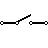switch (closed)cell:battery: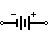diode:resistor:thermistor: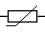27 of 42

## Electrical Circuits: Symbols

variable resistor:LED: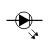lamp:fuse: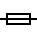voltmeter:ammeter: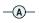28 of 42

## Electrical circuits

f) Current–potential difference graphs are used to show how the current through a component varies with the potential difference across it.
g) The resistance of a component can be found by measuring the current through and potential difference across, the component.
h) The current through a component depends on its resistance. The greater the resistance the smaller the current for a given potential difference across the component.
i) The relationship between potential difference V, current I and resistance R is: V = I x R           j) The current through a resistor (at a constant temperature) is directly proportional to the potential difference across the resistor.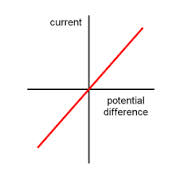29 of 42

## Current and Potential difference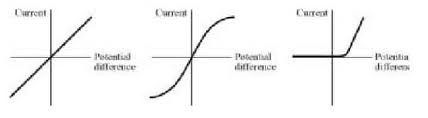j)                                        k)                                    l)

k) The resistance of a filament bulb increases as the temperature of the filament increases.

l) The current through a diode flows in one direction only. The diode has a very high resistance in the reverse direction.

30 of 42

## Current and Potential difference

m) The potential difference provided by cells connected in series is the sum of the potential difference of each cell (depending on the direction in which they are connected).

n) For components connected in series:

• the total resistance is the sum of the resistance of each component
• there is the same current through each component
• the total potential difference of the supply is shared between the components.

o) For components connected in parallel:

• the potential difference across each component is the same
• the total current through the whole circuit is the sum of the currents through the separate components.

31 of 42

## Current and Potential difference

p) An LED emits light when a current flows through it in the forward direction.

Candidates should be aware that the use of LEDs for lighting is increasing, as they use a much smaller current than other forms of lighting.

q) When an electrical charge flows through a resistor, the resistor gets hot.

Candidates should understand that a lot of energy is wasted in filament bulbs by heating. Less energy is wasted in power saving lamps such as Compact Fluorescent Lamps (CFLs). Candidates should understand that there is a choice when buying new appliances in how efficiently they transfer energy

32 of 42

## Household electricity

a) Cells and batteries supply current that always passes in the same direction. This is called direct current (d.c.).

b) An alternating current (a.c.) is one that is constantly changing direction.

c) Mains electricity is an a.c. supply. In the UK it has a frequency of 50 cycles per second (50 hertz) and is about 230 V.

d) A diode may be used for half wave rectification of a.c.

e) Most electrical appliances are connected to the mains using a cable and a three-pin plug.

Candidates should be familiar with the structure of both two-core and three-core cable,with the structure and wiring of a three-pin plug. Knowledge and understanding of the materials used in three-pin plugs is required, as is the colour coding of the covering of the three wires.

33 of 42

## Household electricity

f) If an electrical fault causes too great a current to flow, the circuit is disconnected by a fuse or a circuit breaker in the live wire.

g) When the current in a fuse wire exceeds the rating of the fuse it will melt, breaking the circuit.

h) Some circuits are protected by Residual Current Circuit Breakers (RCCBs), which operate much faster than a fuse.

Candidates should understand that RCCBs operate by detecting a difference in the current between the live and neutral wires.

i) Appliances with metal cases are usually earthed.

Candidates should be aware that some appliances are double insulated, and therefore have no earth wire connection.

j) The earth wire and fuse together protect the wiring of a circuit.

34 of 42

## Household electricity

f) If an electrical fault causes too great a current to flow, the circuit is disconnected by a fuse or a circuit breaker in the live wire.

g) When the current in a fuse wire exceeds the rating of the fuse it will melt, breaking the circuit.

h) Some circuits are protected by Residual Current Circuit Breakers (RCCBs), which operate much faster than a fuse.

Candidates should understand that RCCBs operate by detecting a difference in the current between the live and neutral wires.

i) Appliances with metal cases are usually earthed.

Candidates should be aware that some appliances are double insulated, and therefore have no earth wire connection.

j) The earth wire and fuse together protect the wiring of a circuit.

35 of 42

## Transferring electrical energry

a) The rate at which energy is transferred by an appliance is called the power.

b) The relationship between power P, energy transferred E and time t is:

P= E/t

c) The relationship between power P, current I and potential difference V is:

P  I  V

Candidates should be able to calculate the current through an appliance from its power and the potential difference of the supply and from this determine the size of fuse needed.

d) The relationship between energy transferred E, potential difference V and charge Q is:

E = V x Q

36 of 42

## Transferring electrical energry

e) Everyday electrical appliances are designed to bring about energy transfers.

Candidates should be able to give examples of such devices and energy transfers.

f) The amount of energy an appliance transfers depends on how long the appliance is switched on for and its power.

g) The relationship between energy transferred E from the mains, power P and time t is:

E = P x t

Candidates will not be required to convert between kilowatt-hours and joules. Candidates should be able to calculate the cost of mains electricity given the cost per kilowatt-hour and interpret and use electricity meter readings to calculate total cost over a period of time.

37 of 42

## The National Grid

a) Electricity is distributed from power stations to consumers along the National Grid.

Candidates should be able to identify and label the essential parts of the National Grid.

b) For a given power, increasing the voltage reduces the current required. This reduces the energy losses in the cables.

c) Step-up and step-down transformers are used to change voltages in the National Grid.

Candidates should know why transformers are an essential part of the National Grid.

38 of 42

## Nuclear physics: Atomic Structure

a) The basic structure of an atom is a small central nucleus composed of protons and neutrons surrounded by electrons.

b) The relative masses and relative electric charges of protons, neutrons and electrons are as follows:

Relative mass Relative charge

proton 1 1

neutron 1 0

electron Very small –1

c) In an atom the number of electrons is equal to the number of protons in the nucleus. The atom has no overall electrical charge.

d) Atoms may lose or gain electrons to form charged particles called ions.

39 of 42

## Atomic Structure

e) The atoms of an element always have the same number of protons, but have a different number of neutrons for each isotope.

The total number of protons in an atom is called its proton number or atomic number.

The total number of protons and neutrons in an atom is called its mass number.

Atoms can be represented as shown:

(Mass number) 23

Na

(Atomic number) 11

40 of 42

a) Some substances give out radiation from the nuclei of their atoms all the time, whatever is done to them. These substances are said to be radioactive.

Candidates should be aware of the random nature of radioactive decay.

b) Background radiation is around us all of the time. It comes from:

• natural sources such as rocks and cosmic rays from space
• man-made sources such as the fallout from nuclear weapons testing and nuclear accidents.

c) An alpha particle consists of two neutrons and two protons, the same as a helium nucleus. A beta particle is an electron from the nucleus. Gamma radiation is electromagnetic radiation from the nucleus.

d) Nuclear equations may be used to show single alpha and beta decay.

Candidates will be required to balance such equations, limited to the completion of atomic number and mass number. The identification of daughter elements from such decays is not required.

41 of 42

e) Alpha and beta radiations are deflected by both electric and magnetic fields but gamma radiation is not.

Candidates should know that alpha particles are deflected less than beta particles and in an opposite direction. Candidates should be able to explain this in terms of the relative mass and charge of each particle. Properties of the alpha, beta and gamma radiations are limited to their relative ionising power, their penetration through materials and their range in air.

f) Gamma radiation is not deflected by electric or magnetic fields.

g) There are uses and dangers associated with each type of nuclear radiation.

Candidates should be able to describe the dangers and some uses of each type of radiation.

42 of 42